# 2021 HSC Maths Ext 2 Exam Paper Solutions

The 2021 HSC Maths Extension 2 Exam Paper solutions are here! Read on to see the full worked solutions written by our Head of Mathematics, Oak Ukrit and his team.The wait is over. You can now see how you went with the 2021 HSC Maths Extension 2 Exam Paper!

## 2021 HSC Maths Ext 2 Exam Paper Solutions

Have you seen the 2021 HSC Mathematics Extension 2 Exam Paper yet?

In this post, we will work our way through the 2021 HSC Maths Extension 2 exam paper and give you the solutions, written by our Head of Mathematics, Oak Ukrit and his team. (Doing practice papers? See the solutions for the 2020 HSC Maths Ext 2 Exam here.)

Read on to see how to answer all of the 2021 questions.

## Section 1. Multiple Choice

 Question Number Answer Solution 1. B Since $$\overrightarrow{AB} = \overrightarrow{CD}$$ (both right and flat along the table) and $$\overrightarrow{CQ} = \overrightarrow{DP}$$ (both diagonals across the cube upwards + rightwards + into the page) , we find that $$\overrightarrow{AB}+\overrightarrow{CQ} = \overrightarrow{CD}+\overrightarrow{DP} = \overrightarrow{CP}$$. 2. A Integrate by parts. \begin{align*} \textrm{Let } u &= x^5 \textrm { and } v’ = e^{7x}\\ u’ &= 5x^4 \textrm { and } v = \frac{1}{7}e^{7x}\\ \int x^5 e^{7x} \,dx &= vu – \int u’v \,dx\\ &= \frac{1}{7}e^{7x}x^5 – \int 5x^4\times \frac{1}{7}e^{7x} \,dx\\ &= \frac{1}{7}x^5 e^{7x} – \frac{5}{7}\int x^4 e^{7x} \,dx. \end{align*} 3. B The direction vector needs to be parallel to \begin{align*} \overrightarrow{BA} = \begin{pmatrix} 4 \\ 2 \\5 \end{pmatrix} – \begin{pmatrix} -2 \\ 2 \\ 1 \end{pmatrix} = \begin{pmatrix} 6 \\ 0 \\4 \end{pmatrix}. \end{align*} Out of the 4 options, it is clear that the only direction vector parallel to $$\overrightarrow{BA}$$ is $$(3, 0, 2)$$, hence the solution is \begin{align*} \underset{\text{~}}{r} = \begin{pmatrix} 4 \\ 2 \\5 \end{pmatrix}+\lambda \begin{pmatrix} 3 \\ 0 \\2 \end{pmatrix} \end{align*} 4. C The contrapositive of a statement “if A then B” is “if not B then not A”. We split this statement into parts at the “if” and “then”: \begin{align*} \textrm{For all integers n, } | \textrm{ if } | \textrm{ n is a multiple of 6, } | \textrm{ then } | \textrm{ n is a multiple of 2}. \end{align*} We leave the “for all integers n” at the front, since it is context. Next, we negate the “B” part, i.e. “n is not a multiple of 2”, and also negate the “A” part, i.e. “n is not a multiple of 6”. Finally, we structure as “If Not B then Not A”, and add our context back, to get \begin{align*} \textrm{For all integers n, } | \textrm{ if } | \textrm{ n is not a multiple of 2, } | \textrm{ then } | \textrm{ n is not a multiple of 6}. \end{align*} Check: Remember, the contrapositive must be logically equivalent to the original. If you were asked to prove the original statement, you could equivalently prove “for all integers n, if n is not a multiple of 2, then n is not a multiple of 6”, which is (C). Remark: Observe that (A) is actually the negation! 5. D We can solve this by considering each of the answers: A. The function $$x^3$$ is increasing on its domain. Therefore $$ae^{-b}$$. C. The function $$\ln(x)$$ is increasing on its domain. Therefore $$a 0$$ is a small increment in time. At time $$t+\Delta t$$, the particle has moved slightly further along the curve. Since the speed is increasing, the arrow representing $$\mathbf{v}(t+\Delta t)$$ should be slightly longer than $$\mathbf{v}(t)$$.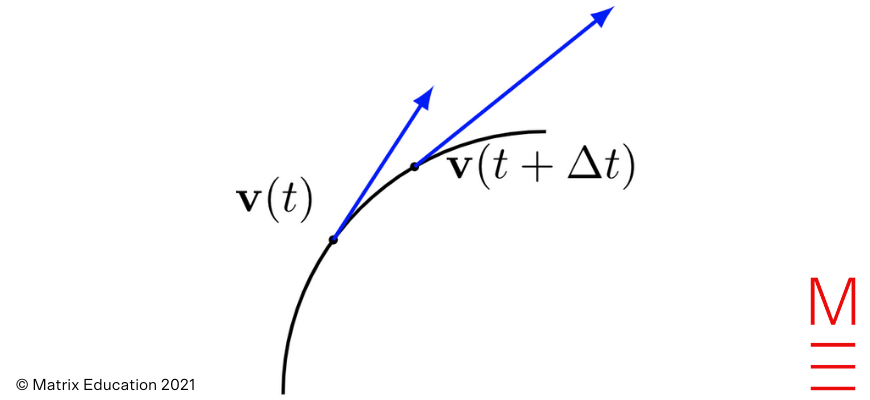Now we consider the difference $$\mathbf{a}(t) \Delta t \approx \mathbf{v}(t+\Delta t) – \mathbf{v}(t)$$: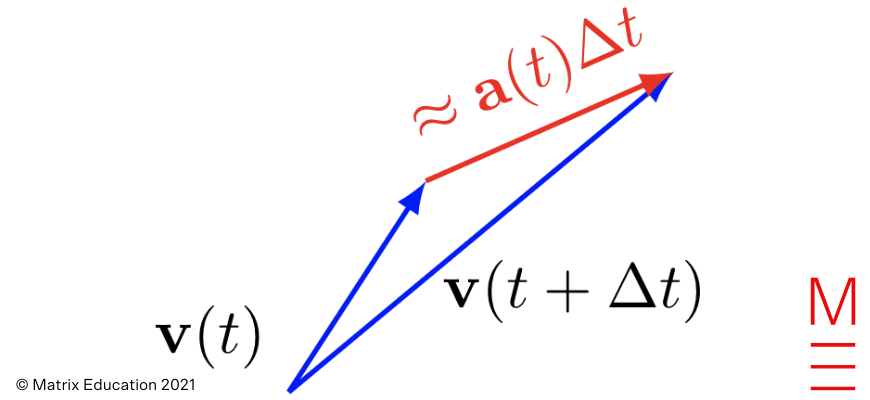The vector $$\mathbf{a(t)}\Delta t$$ is $$\mathbf{a}(t)$$ rescaled by $$\Delta t$$, but the key thing is that they should approximately have the same direction when $$\Delta t$$ is sufficiently small. Consequently we see that (D) is the best match. 9. B This is a classic logic puzzle :). It is best resolved by thinking about the contrapositive, which is always logically equivalent to the original statement: “If a card does not have WIN on one side, then the other side does not have RED”. Sam needs to check both the direct implication and the contrapositive — hence they should flip RED to see if WIN is on the other side, and then flip TRY AGAIN to make sure RED is not on the other side. 10. C If $$z = u+iv$$ and $$w = x+iy$$, then we identify $$z$$ and $$w$$ with the vectors $$z = (u,v)$$ and $$w = (x,y)$$ respectively. The projection of $$z$$ onto $$w$$ is given by \begin{align*} \text{Proj}_w(z) = \frac{z \cdot w}{|w|^2}w. \end{align*} Now observe that $$z \cdot w = ux + vy$$, and $$\text{Re}(zw) = ux – vy$$. Unfortunately this has a minus sign! However, we can easily fix this by considering $$\bar{w}$$ instead: \begin{align*} \text{Re}(z\bar{w}) = ux + vy = z \cdot w. \end{align*} Finally, notice that \begin{align*} \text{Re}\left(\frac{z}{w}\right) = \text{Re}\left(\frac{z\bar{w}}{|w|^2}\right) = \frac{1}{|w|^2}\text{Re}(z\bar{w}) = \frac{z \cdot w}{|w|^2}. \end{align*} This shows that (C) is correct.

Need help with Maths Ext 2?

Expert teachers, weekly quizzes, one-to-one help! Ace your next Maths Ext 2 assessment with Matrix+ Online.

## Section 2. Long Response Questions

Question 11a

We have

 \begin{align*} zw &= 2e^{i\frac{\pi}{2}} \times 6e^{i\frac{\pi}{6}} \\ &=(2 \times 6) e^{i\frac{\pi}{2} + i\frac{\pi}{6}} \\&= 12 e^{i\frac{2\pi}{3}}. \end{align*}

Question 11b

It is easiest to calculate directly:

 \begin{align*} \sum_{n=1}^5 i^n &= i + i^2 + i^3 + i^4 + i^5 \\&= i – 1 – i + 1 + i \\&= i \end{align*}

Question 11c

We have:

 \begin{align*} a \cdot b &= -6 + 0 + 8 = 2\\ |a| &= \sqrt{2^2 + 0^2 + 4^2} = 2\sqrt{5}\\ |b| &= \sqrt{(-3)^2+1^2+2^2} = \sqrt{14} \end{align*}

Thus

 \begin{align*} \cos\theta = \frac{a\cdot b}{|a||b|} = \frac{2}{2\sqrt{5} \sqrt{14}} = \frac{1}{\sqrt{70}} \end{align*}

and $$\theta = \cos^{-1}(\frac{1}{\sqrt{70}}) \approx 83.1^\circ$$.

Question 11d

i) Let $$z=x+iy$$, and $$z^2 = -i$$ so that $$z$$ represents the square roots of $$-i$$.

Expanding, $$z^2 = (x^2-y^2) + 2xyi = -i$$.

Equating real and imaginary parts, $$x^2 = y^2$$ and $$2xy = -1$$.

The first equation gives $$x=\pm y$$. If $$x=y$$, then $$2xy=-1$$ has no real solutions.

Thus $$x=-y$$, and the second equation yields

 \begin{align*} -2x^2 = -1 \Rightarrow x^2 = \frac{1}{2} \Rightarrow x = \pm \frac{1}{\sqrt{2}}. \end{align*}

ii) We use the quadratic formula, with $$a=1$$, $$b=2$$, $$c=1+i$$:

 \begin{align*} z &= \frac{-2 \pm \sqrt{4 – 4(1+i)}}{2}\\ &= -1 \pm \sqrt{-i} \end{align*}

Now, from (i):

 \begin{align*} z&=-1 \pm \sqrt{-i} \\ &= -1 \pm \frac{1}{\sqrt{2}}(1-i) \end{align*}

Thus the two solutions are $$z = (-1+\tfrac{1}{\sqrt{2}}) – \tfrac{1}{\sqrt{2}}i$$ and $$z = -(1+\tfrac{1}{\sqrt{2}}) + \tfrac{1}{\sqrt{2}}i$$.

Question 11e

We start by realising the denominator, by multiplying top and bottom by $$\bar{w}$$:

 \begin{align*} \frac{\bar{z}}{w} &= \frac{\bar{z}\bar{w}}{w\bar{w}}\\ &= \frac{\bar{z}\bar{w}}{|w|^2} \\ &= \frac{(5-i)(2+4i)}{20} \\&= \frac{14+18i}{20} \\&= \frac{7}{10} + \frac{9}{10}i. \end{align*}

Question 11f

Note that the quadratic $$x^2+x+1$$ is irreducible (cannot be factorized) over $$ℝ$$, hence our partial fraction decomposition has the form

 \begin{equation*} \frac{3x^2-5}{(x-2)(x^2+x+1)} = \frac{A}{x-2} + \frac{Bx+C}{x^2+x+1}. \end{equation*}

Multiplying both sides by the denominator, we get

 \begin{equation*} A(x^2+x+1) + (Bx+C)(x-2) \equiv 3x^2-5. \end{equation*}

Setting $$x=2$$ to zero out the second term, we find that

 \begin{align*} A(2^2 + 2 + 1) + (Bx + C)(2-2) &= 3(2^2) + 5\\ 7A &= 7\\ A&=1. \end{align*}

Matching coefficients of $$x^2$$, we find $$A+B=3 \Rightarrow B=2$$. Finally, we match constants to obtain $$A-2C = -5 \Rightarrow C=3$$. Hence

 \begin{align*} \frac{3x^2-5}{(x-2)(x^2+x+1)} = \frac{1}{x-2} + \frac{2x+3}{x^2+x+1}. \end{align*}

Question 12a

Notice that $$x^2 + 2x + 2 = (x+1)^2 + 1$$, so the quadratic cannot be factored over $$ℝ$$, i.e. we cannot use partial fractions.

Instead, we notice that the derivative of the denominator is $$2x+2$$, which leaves 1 in the numerator when separated out:

 \begin{align*} \int \frac{2x+3}{x^2+2x+2} \,dx &= \int \frac{2x+2}{x^2+2x+2} \,dx + \int \frac{1}{x^2+2x+2} \,dx. \end{align*}

The first integral is a logarithm with its derivative on the numerator; the second one is an inverse tan.

 \begin{align*} &= \ln(x^2+2x+2) + \int \frac{1}{(x+1)^2+1} \,dx \\ &= \ln(x^2+2x+2) + \tan^{-1}(x+1) + C. \end{align*}

Question 12b

i) We obtain the converse by swapping the “if” and “then” statements: “if $$n$$ is even, then $$n^2$$ is even”.

ii) Assume that $$n$$ is even, so that $$n=2k$$ for some integer $$k$$. Then $$n^2 = (2k)^2 = 4k^2 = 2(2k^2)$$, hence $$n^2$$ is even.

Question 12c

Since the lines are perpendicular, their direction vectors are perpendicular, and so their dot products are 0:

 \begin{align*} \begin{pmatrix} 1 \\ 0 \\ 2 \end{pmatrix} \cdot \begin{pmatrix} p \\ 3 \\ -1 \end{pmatrix} &=0 \\ p – 2&=0 \\p&=2 \end{align*}

Now we are given that the lines intersect.
Looking at the equation for the $$y$$-coordinate, we see that $$3\mu – 2 = 1 \Rightarrow \mu = 1$$.
Now the $$x$$-coordinate equation gives $$6 = \lambda – 2 \Rightarrow \lambda = 8$$.
Finally, the $$z$$-coordinate equation gives

 \begin{align*} 3+ 16 = q-1 \Rightarrow q = 20. \end{align*}

Question 12d

Firstly, we check the base case on our calculator: $$\sqrt{9!} = 602.3952\ldots$$ and $$2^9 = 512 < \sqrt{9!}$$.
Now assume the statement is true for some $$k\ge 9$$. We need to show that $$2^{k+1} < \sqrt{(k+1)!}$$. Starting with the left hand side of this inequality, we have

 \begin{align*} 2^{k+1} = 2^k \cdot 2 < 2\sqrt{k!} \end{align*}

by assumption.

Now if $$k \ge 9$$, then $$\sqrt{k+1} \ge \sqrt{10} > 2$$. Hence

 \begin{align*} 2^{k+1} < 2\sqrt{k!} < \sqrt{k+1} \sqrt{k!} = \sqrt{(k+1)!} \end{align*}

which completes the induction.

Question 12e

i) Since the diagonals of the square base bisect at $$H$$ we have that $$\overrightarrow{HA} =-\overrightarrow{HC}$$ and $$\overrightarrow{HB}=-\overrightarrow{HD}$$. We then have that

 \begin{align*} \overrightarrow{HA}+\overrightarrow{HB}+\overrightarrow{HC}+\overrightarrow{HD} &= (\overrightarrow{HA}+\overrightarrow{HC})+(\overrightarrow{HB}+\overrightarrow{HD}) \\ &= (-\overrightarrow{HC}+\overrightarrow{HC}) + (-\overrightarrow{HD}+\overrightarrow{HD}) \\ &= \mathbf{0}. \end{align*}

ii)  Starting from the given equation, we want to turn $$\overrightarrow{GA}, \overrightarrow{GB}, \overrightarrow{GC}, \overrightarrow{GD}$$ into $$\overrightarrow{GH}$$.

Consider that for each of A, B, C and D:

 \begin{align*} \overrightarrow{GA} &= \overrightarrow{GH}+\overrightarrow{HA}\\ \overrightarrow{GB} &= \overrightarrow{GH}+\overrightarrow{HB}\\ \overrightarrow{GC} &= \overrightarrow{GH}+\overrightarrow{HC}\\ \overrightarrow{GD} &= \overrightarrow{GH}+\overrightarrow{HD} \end{align*}

Performing these substitutions, we have:

 \begin{align*} \overrightarrow{GA}+\overrightarrow{GB}+\overrightarrow{GC}+\overrightarrow{GD}+\overrightarrow{GS} &= (\overrightarrow{GH}+\overrightarrow{HA})+(\overrightarrow{GH}+\overrightarrow{HB})+(\overrightarrow{GH}+\overrightarrow{HC})+(\overrightarrow{GH}+\overrightarrow{HD})+\overrightarrow{GS}\\ &= (\overrightarrow{HA}+\overrightarrow{HB}+\overrightarrow{HC}+\overrightarrow{HD}) + 4\overrightarrow{GH} +\overrightarrow{GS} \\ &=4\overrightarrow{GH} + \overrightarrow{GS}. \end{align*}

Now, from the question, $$G$$ is such that $$\overrightarrow{GA}+\overrightarrow{GB}+\overrightarrow{GC}+\overrightarrow{GD}+\overrightarrow{GS}=\mathbf{0}$$, so we obtain

 \begin{align*} 4\overrightarrow{GH} + \overrightarrow{GS} = \mathbf{0}. \end{align*}

iii) Using vector addition, we can write $$\overrightarrow{GS} = \overrightarrow{GH}+\overrightarrow{HS}$$.

Substituting into (ii) gives

 \begin{align*} 4\overrightarrow{GH} + \overrightarrow{GS} &= \mathbf{0} \\ 4\overrightarrow{GH} + \overrightarrow{GH}+\overrightarrow{HS} &= \mathbf{0} \\ 5\overrightarrow{GH} &= -\overrightarrow{HS} \\ \overrightarrow{GH} &= -\frac{1}{5} \overrightarrow{HS} \\ \overrightarrow{HG} &= \frac{1}{5}\overrightarrow{HS} \end{align*}

so we conclude that $$\lambda = \frac{1}{5}$$.

Question 13a

Each of the 4th roots has the same magnitude, and are rotated by $$\frac{2\pi}{4} = 90\text{˚}$$. Since the magnitude of $$a+ib$$ as indicated is greater than 1, our resultant magnitude should also be greater than 1 (i.e. outside the unit circle), but smaller than the original. Additionally, we use the lines provided to draw a vector with a quarter of the modulus; then we rotate it 4 times by $$90 \text{˚}$$, giving us our solution as indicated in pink below: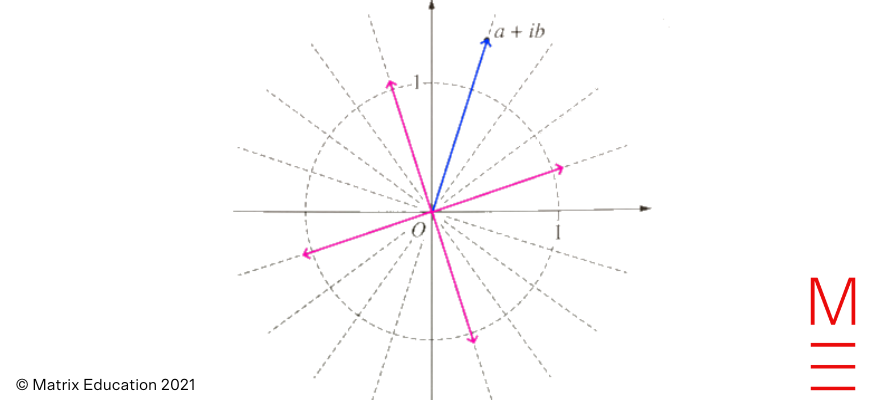Question 13b

The most straightforward substitution is $$u = \sqrt{x^2 – 9}$$. This gives:

 \begin{equation*} du = \frac{x}{\sqrt{x^2 – 9}} \,dx \Rightarrow u\,du = x\,dx. \end{equation*}

When $$x=\sqrt{10}$$ and $$\sqrt{13}$$, we have $$u=1$$ and 2 respectively. Therefore

 \begin{align*} \int_{\sqrt{10}}^{\sqrt{13}} x^3 \sqrt{x^2 – 9} \,dx &= \int_{\sqrt{10}}^{\sqrt{13}} x^2 \sqrt{x^2 – 9} \,(x\,dx) \\ &= \int_1^2 (u^2+9)u^2 \,du \\ &= \int_1^2 u^4 + 9u^2 \,du \\ &= \left[ \frac{u^5}{5} + 3u^3 \right]^2_1 \\ &= \left(\frac{32}{5}+24\right) – \left(\frac{1}{5}+3\right) \\ &= \frac{136}{5}. \end{align*}

i) We use integration by parts with $$u = (\ln x)^n$$ and $$dv = 1$$. Thus $$du = n(\ln x)^{n-1} \frac{1}{x}$$ and $$v=x$$. Using these parts, we can derive the reduction formula from the question:

 \begin{align*} I_n = \int_1^e (\ln x)^n \,dx &= \bigg[(\ln x)^n x \bigg]^e_1 – \int_1^e n(\ln x)^{n-1} \,dx \\ &= e – nI_{n-1} \qquad \text{for all } n\ge 1. \end{align*}

ii) We find $$V_B$$ first, which is found by subtracting the volume of the solid of revolution under $$y=\ln x$$ from $$x=1$$ to $$x=e$$ from the volume of the cylinder with radius $$1$$ and height $$e-1$$:

 \begin{align*} V_B &= \pi (e-1) – \pi \int_1^e (\ln x)^2 \,dx \\ &= \pi (e-1) – \pi I_2 \\ &= \pi (e-1) – \pi(e – 2I_1) \\ &= 2\pi I_1 – \pi. \end{align*}

using part (i). Using the reduction formula again and noting that $$I_0 = e-1$$, we have

 \begin{equation*} 2\pi I_1 – \pi = 2\pi(e – I_0) – \pi = 2\pi(e – (e-1)) – \pi = \pi \end{equation*}

and therefore $$V_B = \pi$$. Now $$V_A$$ can be found by subtracting the volume of half a solid sphere of radius 1 from the volume of a cylinder of height 1 and base radius 1. Hence

 \begin{equation*} V_A = \pi – \frac{1}{2}\times\frac{4}{3}\pi = \frac{\pi}{3} = \frac{1}{3}V_B. \end{equation*}

Question 13c

i) Noting that $$\ddot x = -4(x-3)$$, we identify that $$n=2$$ and the centre of the motion is $$x_0=3$$.

Using the relationship

 \begin{equation*} v^2 = n^2\left(A^2-(x-x_0)^2\right) \end{equation*}

and the fact that when $$x=0$$, $$v^2=64$$ we may solve for the amplitude of the motion through

 \begin{align*} 64 &= 4(A^2-9) \\ 16 &= A^2-9 \\ A^2 &= 25 \\ A &= 5 \qquad (A>0). \end{align*}

We have that

 \begin{equation*} x_0 – A \leq x \leq x_0 + A \Longrightarrow -2 \leq x \leq 8 \end{equation*}

and so the particle oscillates between the values $$x=-2$$ and $$x=8$$.

ii) We first model the motion of the particle, then solve for $$x=0$$.

Since the particle starts moving back towards the origin (cosine) rather than towards the endpoint (sine), we can let for the displacement-time equation be

 \begin{equation*} x = x_0 + A \cos(nt+\alpha) \end{equation*}

where $$x_0=3,A=5,n=2$$ and $$0<\alpha<\frac{\pi}{2}$$. So we have

 \begin{equation*} x = 3 + 5 \cos (4t + \alpha) \end{equation*}

We now substitute the condition that $$x(0)=5.5$$ to find $$\alpha$$:

 \begin{align*} 5.5 &= 3 + 5\cos\alpha \\ 5 \cos \alpha &= 2.5 \\ \cos \alpha &= \frac{1}{2} \\ \alpha &= \frac{\pi}{3} \end{align*}

Finally, solving for the first $$t>0$$ for which $$x=0$$:

 \begin{align*} 3 + 5 \cos\left(4t+ \frac{\pi}{3}\right) &= 0 \\ \cos\left(4t + \frac{\pi}{3}\right) &= -\frac{3}{5} \\ 4t + \frac{\pi}{3} &= \cos^{-1}\left(-\frac{3}{5}\right) \\ t &= \frac{1}{4} \left( \cos^{-1}\left( -\frac{3}{5}\right) – \frac{\pi}{3}\right) \\ &\approx 0.29 \text{ correct to 2 decimal places.} \end{align*}

Question 14a

We use the $$t$$-substitution $$t=\tan\left(\frac{x}{2}\right)$$ where $$\mathrm{d}x = \frac{2}{1+t^2} \ \mathrm{d}t$$. When $$x=0,t=0$$ and when $$x=\frac{\pi}{2}, t=1$$. Evaluating the integral we get:

 \begin{align*} \int_0^{\pi/2} \frac{1}{3+5\cos x} \ \mathrm{d}x &= \int_0^1 \frac{1}{3+5\frac{1-t^2}{1+t^2}} \cdot \frac{2}{1+t^2} \ \mathrm{d}t \\ &= \int_0^1 \frac{2}{3(1+t^2) + 5(1-t^2)} \ \mathrm{d}t \\ &= \int_0^1 \frac{1}{4-t^2} \ \mathrm{d}t \\ &= \int_0^1 \frac{1}{(2-t)(2+t)} \ \mathrm{d}t \\ &= \frac{1}{4} \int_0^1 \frac{1}{2-t} + \frac{1}{2+t} \ \mathrm{d}t \\ &= \frac{1}{4} \left[\ln \left| \frac{2+t}{2-t}\right| \right]_0^1 \\ &= \frac{\ln{3}}{4}. \end{align*}

Question 14b

i) Consider the diagram below: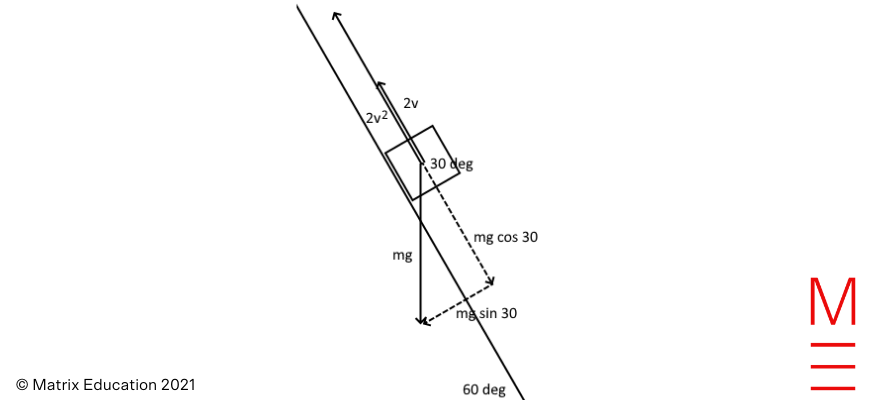Resolving the gravity force of $$5g$$ down the slope, we have that gravity contributes to a force of $$5g \cos(30^\circ)$$ down the slope. We have the component of gravity equal to $$5g \cos(30^\circ)=\frac{5\sqrt{3}}{2} g$$ and two opposing forces $$2v$$ and $$2v^2$$. Hence the resultant force down the slope is given by

 \begin{equation*} \frac{5\sqrt{3}}{2}g – 2v -2v^2. \end{equation*}

ii) For the object to slide down the slope at constant speed, we have that the acceleration is zero. Using Newton’s second law, we have that

 \begin{equation*} 5a = \frac{5\sqrt{3}}{2}g – 2v -2v^2. \end{equation*}

Setting $$a=0$$ and $$g=10$$ gives the quadratic:

 \begin{equation*} 2v^2 + 2v – 25\sqrt{3}=0 \end{equation*}

 \begin{equation*} v = \frac{-2 + \sqrt{4+200\sqrt{3}}}{4} \approx 4.2 \mathrm{ms}^{-1} \end{equation*}

where we take the positive square root as $$v$$ is directed down the slope.

Question 14c

i) Using De Moivre’s Theorem, we have that

 \begin{equation*} (\cos\theta+i\sin\theta)^5 = \cos5\theta + i \sin5\theta \end{equation*}

However by the binomial theorem, we have that

 \begin{align*} (\cos\theta+i\sin\theta)^5 &= \cos^5\theta+5\cos^4\theta (i\sin\theta) – 10\cos^3\theta \sin^2\theta -10\cos^2\theta (i\sin^3\theta) + 5 \cos\theta \sin^4\theta + i\sin^5\theta \\ &= (\cos^5\theta- 10\cos^3\theta \sin^2\theta+5 \cos\theta \sin^4\theta) + i(\ldots\text{imaginary part}\ldots). \end{align*}

Equating the real parts of both these expressions for $$(\cos\theta+i\sin\theta)^5$$ we obtain

 \begin{equation*} \cos5\theta = \cos^5\theta- 10\cos^3\theta \sin^2\theta+5 \cos\theta \sin^4\theta \end{equation*}

To express this as a polynomial in $$\cos \theta$$ only, we use the identity $$\sin^2\theta = 1- \cos^2\theta$$ to obtain

 \begin{align*} \cos5\theta &= \cos^5\theta- 10\cos^3\theta \sin^2\theta+5 \cos\theta \sin^4\theta \\ &= \cos^5\theta- 10\cos^3\theta (1-\cos^2\theta)+5 \cos\theta (1-\cos^2\theta)^2 \\ &= \cos^5\theta -10\cos^3\theta+10\cos^5\theta +5\cos\theta(1-2\cos^2\theta+\cos^4\theta) \\ &= 16\cos^5\theta – 20 \cos^3\theta +5 \cos\theta \end{align*}

as required.

ii) Note that

 $$\text{Re}(e^{i\frac{\pi}{10}}) = \text{R}e(\cos\frac{\pi}{10}+i\sin\frac{\pi}{10})=\cos\frac{\pi}{10}$$.

Let $$\theta = \frac{\pi}{10}$$; then, using (i):

 \begin{align*} 16\cos^5{\theta} – 20\cos^3{\theta} + 5\cos{\theta} &= \cos\left(\frac{5\pi}{10}\right)=0. \end{align*}

If we now let $$x=\cos{\theta}$$, we can write:

 \begin{align*} 16x^5 – 20x^3 + 5x &= 0 \\ 16x^4 – 20x^2 + 5 &= 0 \end{align*}

where we note that $$x\neq0$$, making it valid to divide both sides by $$x$$. Treating this as a quadratic equation in $$x^2$$ we obtain

 \begin{align*} x^2 &= \frac{-(-20)\pm\sqrt{(20)^2 – 4\times 16\times 5}}{2\times16} \\ &= \frac{20\pm\sqrt{80}}{32} \\ &= \frac{5\pm\sqrt{5}}{8} \\ x &= \pm \sqrt{\frac{5\pm\sqrt{5}}{8}}. \end{align*}

Now, $$\cos\frac{\pi}{10}>0$$ as it is in the first quadrant; so we have

 \begin{align*} x &= \sqrt{\frac{5\pm\sqrt{5}}{8}} = 0.951 \textrm{ or } 0.5877. \end{align*}

where 0.951 is taking $$+\sqrt{5}$$ and 0.5877 is taking $$-\sqrt{5}$$.

For the inner sign, we note that $$0<\frac{\pi}{10}<\frac{\pi}{4}$$, and so

 \begin{equation*} 0.707\approx \cos \frac{\pi}{4} < \cos \frac{\pi}{10} < \cos 0 = 1 \end{equation*}

and hence we must choose the inner $$+$$ sign to obtain $$x \approx 0.951$$ since $$0.5877$$ is too small. We can now conclude that

 \begin{equation*} \cos \frac{\pi}{10} = \sqrt{ \frac{5+\sqrt{5}}{8} }. \end{equation*}

Question 15a

i) We are given $$\sqrt{xy} \le \frac{x+y}{2}$$.

We begin by substituting $$x=ab$$ and $$y=c$$ to get the LHS:

 \begin{align*} \sqrt{xy} &\le \frac{x+y}{2}\\ \sqrt{(ab)c} &\le \frac{ab+c}{2} \end{align*}

Next, we substitute $$x=a^2$$ and $$y=b^2$$ to eliminate the ab term on our RHS:

 \begin{align*} \sqrt{a^2b^2} &\le \frac{a^2+b^2}{2}\\ ab &\le \frac{a^2+b^2}{2}. \end{align*}

Substituting, we have:

 \begin{align*} \sqrt{(ab)c} &\le \frac{ab+c}{2}\\ &\le \frac{\frac{a^2+b^2}{2}+c}{2}\\ &= \frac{a^2+b^2+2c}{4} \end{align*}

as required.

ii) The key observation is that the left hand side of the inequality in (i) is unchanged if the labels $$a,b,c$$ are swapped with each other. Hence we obtain 2 other inequalities for a total of 3:

 \begin{align*} \sqrt{abc} &\le \frac{a^2+b^2+2c}{4} \\ \sqrt{abc} &\le \frac{b^2+c^2+2a}{4} \\ \sqrt{abc} &\le \frac{a^2+c^2+2b}{4}. \end{align*}

Adding up the inequalities just obtained, we find

 \begin{align*} 3\sqrt{abc} &\le \frac{2(a^2+b^2+c^2)+2(a+b+c)}{4} \\ \sqrt{abc} &\le \frac{(a^2+b^2+c^2)+(a+b+c)}{6} \end{align*}

as required.

Question 15b

i) The question asks us to prove that every odd triangular number is a hexagonal number.

Let $$n=2k+1$$ be an odd integer, where $$k$$ is an integer $$\ge 0$$. Then

 \begin{align*} t_n &= \frac{n(n+1)}{2} \\ &= \frac{(2k+1)(2k+2)}{2} \\ &= (k+1)(2k+1). \end{align*}

Now note that the $$m$$-th hexagonal number is given by $$h_m = 2m^2 – m = m(2m-1)$$. If we set $$m = k+1$$ to match the brackets, then $$2m-1= 2(k+1)-1 = 2k+1$$, so we can write:

 \begin{align*} t_{2k+1} &= (k+1)(2k+1) \\ &= m(2m-1)\\ &= 2m^2 – m \\ &= h_m. \end{align*}

Thus the $$(2k+1)$$-th triangular number is the $$(k+1)$$-th hexagonal number; or put another way, the $$(2k-1)$$-th triangular number is the $$k$$-th hexagonal number.

ii) We note that in the previous step, we’ve mapped each hexagonal number one-to-one with each triangular number; i.e. $$h_1 = t_3$$, $$h_2 = t_5$$, $$h_m = t_{2m-1}$$.

Suppose $$t_{2k}$$, an even triangular number, was a hexagonal number $$h_n$$.

We know that triangular numbers are increasing, so $$t_{2k-1}<t_{2k}<t_{2k+1}$$.

Since each of the surrounding odd triangular numbers is a hexagonal number, then $$h_{k}<t_{2k}<h_{k+1}$$.

However, hexagonal numbers are also increasing; and only make sense for integer coefficients. Therefore, there cannot be a hexagonal number $$h_n = t_{2k}$$ such that $$h_{k}<h_n<h_{k+1}$$, so $$t_{2k} \neq h_n$$.

Question 15c

i) Using Newton’s second law we have the equation

 \begin{align*} m \ddot x &= -mg – kv^2 \ (\textrm{where } m =1)\\ \ddot x &= -(g+kv^2) \end{align*}

Next, we use the relationship $$\ddot x = \frac{dv}{dt}$$ to find $$T$$ when $$v=0$$ at the maximum height of the object, with limits $$t=0,v=u$$ and $$t=T,v=0$$:

 \begin{align*} \frac{dv}{dt} &= -(g+kv^2) \\ \int_u^0 \frac{1}{k\left(\frac{g}{k}+v^2\right)} \ \mathrm{d}v &= – \int_0^{T} \ \mathrm{d}t \\ \frac{1}{k}\sqrt{\frac{k}{g}}\left[\tan^{-1}\left(u\sqrt{\frac{k}{g}}\right)\right]_u^0 &= – T \\ \frac{1}{\sqrt{gk}}\left(0-\tan^{-1}\left(u\sqrt{\frac{k}{g}}\right)\right) &= – T \\ \end{align*}

We obtain that the time taken to reach the maximum height is

 \begin{equation*} T = \frac{1}{\sqrt{gk}} \tan^{-1}\left(u\sqrt{\frac{k}{g}}\right). \end{equation*}

ii) This time we use the relationship $$\ddot x = v \frac{\mathrm{d}v}{\mathrm{d}x}$$, where we have that $$v=0$$ at the maximum height. Using these conditions, we integrate with limits $$x=0,v=u$$ at the bottom and $$x=H, v=0$$ at the maximum height:

 \begin{align*} v \frac{\mathrm{d}v}{\mathrm{d}x} &= – (g+ kv^2) \\ \int_u^0 \frac{v}{g+kv^2} \ \mathrm{d}v &= – \int_0^H \ \mathrm{d}x \\ \frac{1}{2k} \int_u^0 \frac{2kv}{g+kv^2} \ \mathrm{d}v &= – \int_0^H \ \mathrm{d}x \\ \frac{1}{2k} \left[\ln(g+kv^2)\right]_u^0 &= – H \\ \frac{1}{2k} \ln \left( \frac{g}{g+ku^2}\right) &= -H \\ H&= \frac{1}{2k} \ln \left( \frac{g+ku^2}{g}\right). \end{align*}

We obtain the maximum height as

 \begin{equation*} H = \frac{1}{2k} \ln \left( 1 + \frac{ku^2}{g} \right). \end{equation*}

Question 15d

For $$n=2$$, observe that $$2^2 + 3^2 = 13 < 25 = 5^2$$. We claim that $$2^n + 3^n < 5^n$$ for all $$n \ge 2$$. The base case $$n=2$$ has just been verified. Now, assuming the claim to be true for some $$n \ge 2$$, we observe that

 \begin{align*} 2^{n+1} + 3^{n+1} &= 2^n \times 2 + 3^n \times 3 \\ &< 3(2^n + 3^n) \\ &< 5^n \times 3 \\ &< 5^{n+1}. \end{align*}

Thus the statement is true for $$n+1$$. Hence our claim holds true by the principle of mathematical induction. Alternatively we could use a binomial expansion, and note that

 \begin{equation*} 5^n = (2+3)^n = 2^n +3^n +\sum_{k=1}^{n-1} \binom{n}{k} 2^{n-k} 3^k > 2^n + 3^n \ \text{for all } n \geq2. \end{equation*}

Question 16a

i) Since $$\mathbf{i}, \mathbf{j}, \mathbf{k}$$ are all unit vectors, the triangle inequality implies

 \begin{equation*} 1 = |\overrightarrow{OP}|=|x\mathbf{i} + y\mathbf{j} + z\mathbf{k}| \le |x\mathbf{i}| + |y\mathbf{j}| + |z\mathbf{k}|= |x|+|y|+|z| \end{equation*}

where $$|\overrightarrow{OP}|=1$$ since $$\overrightarrow{OP}$$ is a position vector of a point on the unit sphere. From the above, we obtain that

 \begin{equation*} |x|+|y|+|z| \ge 1 \end{equation*}

ii) Recalling the geometric dot product between vectors $$\mathbf{a}$$ and $$\mathbf{b}$$ we have that

 \begin{equation*} |\mathbf{a}\cdot \mathbf{b}| = ||\mathbf{a}| |\mathbf{b}| \cos \theta | \leq |\mathbf{a}| |\mathbf{b}| \end{equation*}

since $$0\leq |\cos \theta| \leq 1$$. Substituting for $$\mathbf{a}$$ and $$\mathbf{b}$$ gives

 \begin{equation*} |a_1 b_1 + a_2 b_2 + a_3 b_3 | \leq \sqrt{a_1^2+a_2^2+a_3^2} \sqrt{b_1^2+b_2^2+b_3^2} \end{equation*}

as required.

iii) Consider the inequality in (ii) with the vectors $$\mathbf{a} = (1,1,1)$$ and $$\mathbf{b} =(|x|,|y|,|z|)$$. We immediately obtain

 \begin{equation*} |x|+|y|+|y| \leq \sqrt{1+1+1} \sqrt{|x|^2+|y|^2+|z|^2} = \sqrt{3}\sqrt{x^2+y^2+z^2} = \sqrt{3}. \end{equation*}

Remark: We note that a more general version of this question appeared in the Matrix Term 2 Workbook in 2020 :).

Question 16b

We have that the position vector is

 \begin{equation*} \underset{\text{~}}{r}(t) = \begin{pmatrix} ut\cos\theta \\ ut\sin\theta – \frac{1}{2}gt^2 \end{pmatrix} \end{equation*}

We differentiate each term with respect to time to get the velocity vector:

 \begin{equation*} \underset{\text{~}}{v}(t) = \begin{pmatrix} u\cos\theta \\ u\sin\theta -gt \end{pmatrix}. \end{equation*}

In two perpendicular vectors, as in two perpendicular lines, the gradients multiply to get -1:

 \begin{align*} \frac{u\sin\theta-gt}{u\cos\theta} \times \frac{ut\sin\theta-\frac{1}{2}gt^2}{ut\cos\theta} &= -1 \end{align*}

Multiplying both sides of the equation by $$2u^2\cos^2\theta$$ gives

 \begin{align*} (u\sin\theta-gt)(2u\sin\theta-gt)&=-2u^2\cos^2\theta \\ 2u^2\sin^2\theta-(3gu\sin\theta)t+g^2t^2 &= -2u^2(\cos^2\theta) \\ g^2t^2 – (3gu\sin\theta)t+ 2u^2\sin^2\theta + 2u^2 cos^2 \theta&= 0 \\ g^2t^2 -(3gu\sin\theta) t+2u^2 &= 0 \end{align*}

where we use the fact that $$\sin^2\theta+\cos^2\theta=1$$ in the last step. Observe that this is a quadratic in $$t$$ with discriminant

 \begin{equation*} \Delta = (-3gu\sin\theta)^2 – 4(g^2)(2u^2) = 9u^2g^2\sin^2\theta – 8u^2g^2. \end{equation*}

To get two solutions for $$t$$, we solve $$\Delta > 0$$ to obtain

 \begin{align*} 9u^2g^2\sin^2\theta – 8 u^2g^2 &> 0 \\ 9u^2g^2\sin^2\theta &> 8 u^2g^2 \\ \sin^2\theta &> \frac{8}{9} \\ \sin \theta &> \frac{2\sqrt{2}}{3}. \end{align*}

Finally, considering the particle cannot be projected at an angle more than 90 degrees (vertically upwards), we have

 \begin{equation*} \sin^{-1}\left(\frac{2\sqrt{2}}{3}\right) < \theta < \frac{\pi}{2}. \end{equation*}

Once the angle $$\theta$$ is determined, we must also check that these two solutions for $$t$$ physically make sense, i.e. that they are between $$0$$ and $$\frac{2u \sin \theta}{g}$$ (the time of flight). Solving for $$t$$ would give

 \begin{align*} t &= \frac{1}{2} \left( \frac{3u\sin\theta}{g} \pm \sqrt{ \left(\frac{u}{g}\right)^2 (9 \sin^2 \theta – 8)}\right) \\ &= \frac{u}{2g} \left(3\sin\theta \pm \sqrt{9\sin^2\theta-8}\right). \end{align*}

Both of the plus and minus variants are positive: the minus variant is positive since

</tableand so
The larger solution is less than the flight time since
Question 16cWe consider each quadrant separately.Quadrant 1: In this quadrant, $$x\geq0,y\geq0$$ and $$0\leq \text{Arg}(z) \leq \frac{\pi}{2}$$. We would then be solving the inequality:where we note that this region need only be sketched on $$0\leq x \leq \frac{\pi}{2}$$ since it is trivially always true for $$x > \frac{\pi}{2}$$.
Quadrant 2:  Since $$x<0$$ but $$\frac{\pi}{2} \leq \text{Arg}(z) \leq \pi$$ then it never be the case that $$\text{Re}(z)\geq \text{Arg}(z)$$
Quadrant 3: We have thatolving the inequality:where multiplying both sides by $$x<0$$ in the last step reverses the direction of the inequality. Noting that we only sketch this region for $$-\pi \leq x \leq -\frac{\pi}{2}$$ and then there will be no solution for $$x < -\pi$$ and all solutions for $$x>-\frac{\pi}{2}$$.
Quadrant 4: We have that $$x>0$$ but $$\text{Arg}(z)<0$$ and so all of Quadrant 4 will be part of the region.
Finally at $$z=0$$, $$\text{Arg}(z)$$ is undefined so we have an open circle at the origin.In total we have the following plot: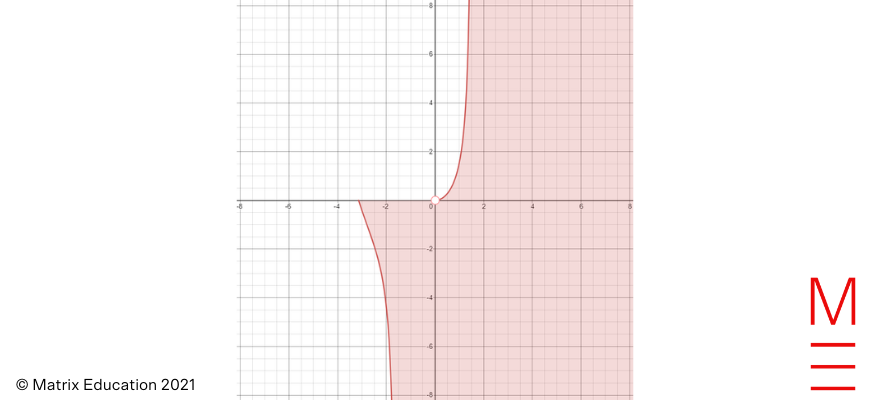$$\sqrt{9\sin^2\theta-8} < \sqrt{9\sin^2\theta} < 3 \sin\theta$$ $$t > \frac{u}{2g}(3\sin\theta-\sqrt{9\sin^2\theta}) = 0$$ \begin{align*} t &= \frac{u}{2g} \left(3\sin\theta + \sqrt{9\sin^2\theta-8}\right) \\ &< \frac{u}{2g} \left(3\sin\theta + \sqrt{9\sin^2\theta-{\color{red}{8\sin^2\theta}}}\right) \\ &= \frac{2u\sin\theta}{g}. \end{align*} \begin{align*} x &\geq \tan^{-1}\left(\frac{y}{x}\right) \\ \tan x &\geq \frac{y}{x} \\ y &\leq x \tan x \end{align*} $$\text{Arg}(z) = -\pi + \tan^{-1}\left| \frac{y}{x} \right| = -\pi + \tan^{-1}\left(\frac{y}{x}\right)$$. \begin{align*} x &\geq – \left( \pi – \tan^{-1}\left(\frac{y}{x}\right) \right) \\ \tan^{-1}\left(\frac{y}{x}\right) &\leq x + \pi \\ \frac{y}{x} &\leq \tan(x+\pi) \\ y &\geq x \tan(x+\pi), \end{align*}

Need help with Maths Ext 2?

Expert teachers, weekly quizzes, one-to-one help! Ace your next Maths Ext 2 assessment with Matrix+ Online.